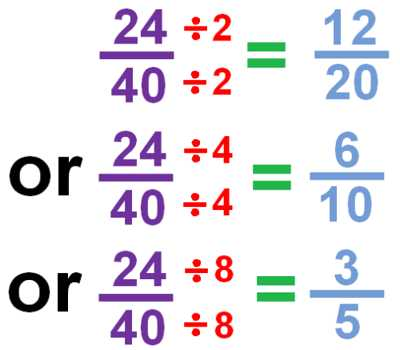Simplifying Fractions

Fractions may have numerators and denominators that are composite numbers (numbers that have more factors than 1 and itself). Simplifying (or reducing) fractions means to make the fraction as simple as possible.

How to simplify a fraction:

• Find a common factor of the numerator and denominator. A common factor is a number that will divide into both numbers evenly. Two is a common factor of 4 and 14.
• Divide both the numerator and denominator by the common factor.
• Repeat this process until there are no more common factors.
• The fraction is simplified when no more common factors exist.

Steps to simplifying a proper fraction: Example: 12/20

Step 1: Determine the factors of the numerator. The factors of 12 are 1, 2, 3, 4, 6 and 12.

Step 2: Determine the factors of the denominator. The factors of 20 are: 1, 2, 4, 5, 10, 20.

Step 3: Find the greatest common factor. The greatest common factor is 4

Step 4: Divide both the numerator and the denominator by the greatest common factor. 12 divided by 4 is 3. 20 divided 4 is 5.When reducing fractions by hand it may be easier to repeatedly divide numerator and denominator by factors that are common to both of them. The process is complete when the numerator and the denominator have no more factors in common.

Reduced fractions are fractions that have been reduced to their simplest form. When the GCF of the numerator and denominator is equal to 1, the fraction cannot be reduced any further.

Another method to simplify a fraction

• Find the Greatest Common Factor (GCF) of the numerator and denominator
• Divide the numerator and the denominator by the GCF.

Information Source: